Previous: Merging Paths  Up: Selecting and Editing Objects  Next: Exclusive Or Function

# Path Union

Multiple shapes can be combined into a single shape by performing a union on all the selected shapes using the menu item Transform->Path Union. At least two shapes must be selected to perform this function. As with the merge path function, the new shape has the same styles as the backmost path in the selection.

For example, in Figure 8.35(a), there are three overlapping paths. In Figure 8.35(b) the paths have been replaced by a single path created using the path union function. For comparison, the same three paths in Figure 8.35(a) were replaced using the merge function. The result is shown in Figure 8.35(c).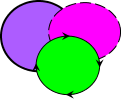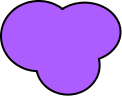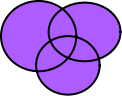(a) (b) (c)
Figure 8.35: Path union: (a) original paths (the rear path has an orchid fill colour, 2bp line width and round join style); (b) the three paths in (a) have been replaced by a single path using the path union function; (c) for comparison, the three paths in (a) have been replaced by a single path using the merge paths function.

In Figure 8.36, a text-path and a path are combined: Figure 8.36(a) shows the original objects and Figure 8.36(b) shows the resulting object. In this case, the resulting object is a text-path since the backmost path in Figure 8.36(a) was the text-path object.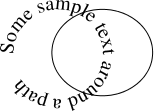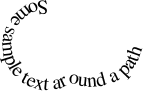(a) (b)
Figure 8.36: Text-path union: (a) original text-path and path; (b) objects in (a) have been replaced with text-path.

Any pattern in the selection will first be converted to a full path before the union is applied. For example, in Figure 8.37, two patterns are combined: Figure 8.37(a) shows the original patterns (both have a rotational pattern with 2 replicas) and Figure 8.37(b) shows the resulting path. This path is illustrated in edit mode in Figure 8.37(c) to show that it is now a full path.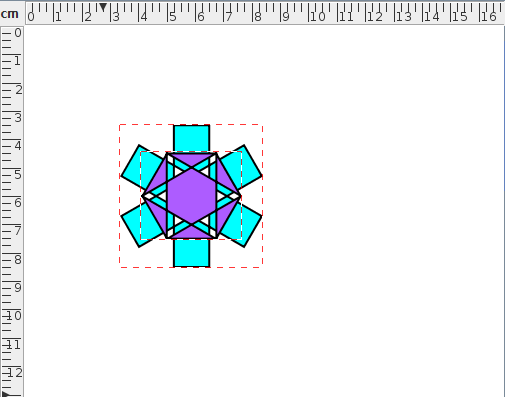(a)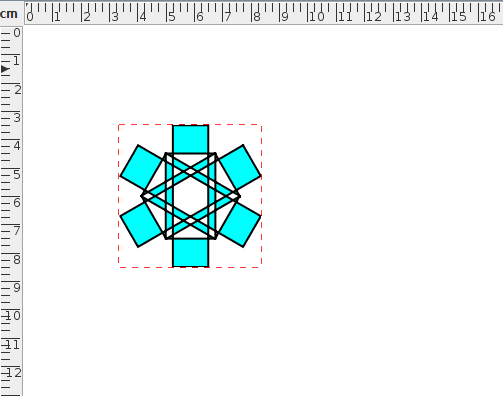(b)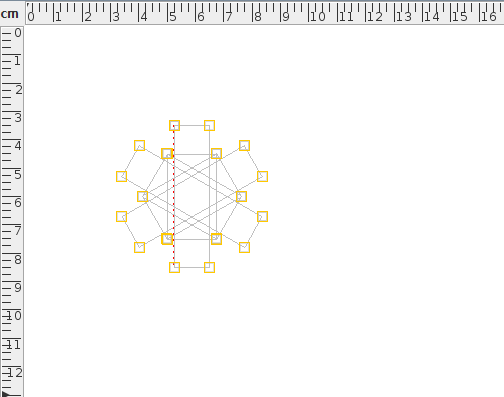(c)
Figure 8.37: Pattern union: (a) original patterns; (b) patterns in (a) have been combined to form a full path; (c) result shown in edit mode.